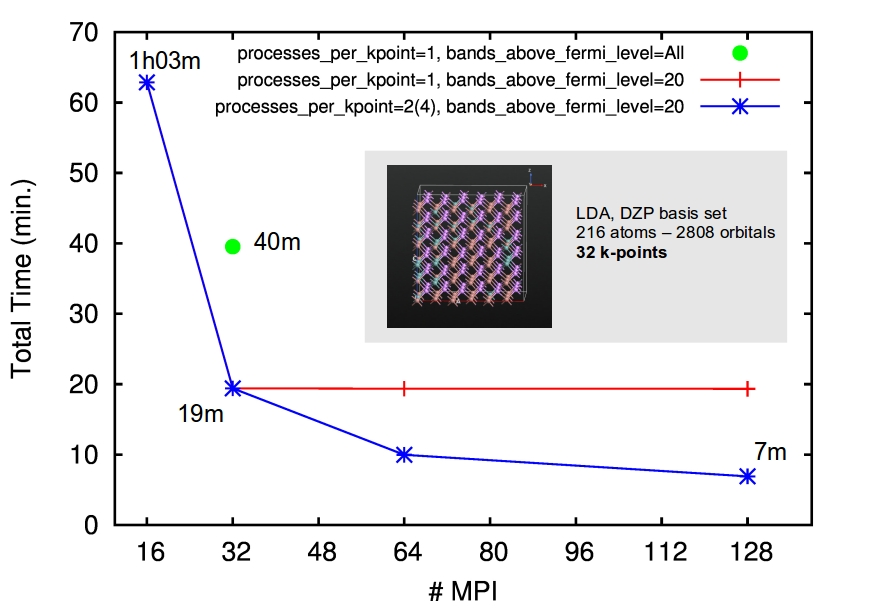# Performance troubleshooting guide¶

In this tutorial, you will learn which are the main parameters to tune for improvimg ATK performance for various systems. In order to get the highest possible performance of your calculations, ATK provides an array of different algorithms and utilizes both MPI and shared-memory threading for parallelization. As already mentioned in the technical note Parallelization of QuantumATK calculations, MPI parallelization will ususlly give the best speedup of calculations, while threading of processes can often reduce the memory footprint.

Getting the absolute peak performance out of any high performance software requires somewhat deep knowledge of both the calculation in question, and the hardware used. It is therefore important to optimize your QuantumATK simulation with respect to hardware used and parallelization method. However, the following sections give some tips for QuantumATK algorithms and options that may also help in fixing issues with memory or speed.

## Running out of memory?¶

General indications

• Try to disable store_grids in AlgorithmParameters, this will lower the memory slightly, and will reduce the calculation time very little.
• Disabling store_basis_on_grid in AlgorithmParameters will reduce the memory significant, and if the matrix size of the system is small, it can have negative effect on the performance. This parameter is by default turned off, if the configuration is large, so it might already be disabled. If running in parallel, the memory reduction will be lower per core, since the memory is distributed.
• If running in parallel with more than two processers, try setting the algorithm in IterationControlParameters to ParallelPulayMixer.

Specific for molecules and bulk configurations

• If the matrix size is very large, consider setting density_matrix_method in AlgorithmParameters to DiagonalizationSolver(processes_per_kpoint=2). This will reduce the memory usage per mpi-process by a factor of 2. Try to increase processes_per_kpoint, if the problem persist.
• If the calculation is very large, and the calculation is run in parallel over many nodes, it can be worth trying setting density_matrix_method in AlgorithmParameters to ChebyshevExpansionSolver. When run in massive parallel, this will reduced the memory usage dramatically, however it is also slow compared to ordinary routines.

Specific for device configurations

• Try setting storage_strategy in SelfEnergyCalculator to StoreOnDisk or NoStorage. This will reduce the memory significantly, but will also cost performance for the latter. If running in parallel, the memory reduction will be lower per core, since the memory is distributed.
• The device has a wide cross section, try setting equilibrium_method and/or non_equilibrium_method to SparseGreensFunction
• If run in parallel, setting equilibrium_method and/or non_equilibrium_method to SparseGreensFunction(processes_per_contour_point=2) will help. If the problem persist, try setting processes_per_contour_point=4.

## Want to make it run faster?¶

General indications

• If the calculation requires the usage of the multigrid method, as in the presence of gates, it is possible to consider DirectSolver for the possion_solver. It will require significant more memory, but the overhead is memory distributed so if running in parallel it can be worth to try it.
• Set store_basis_on_grid in AlgorithmParameters to True. This will make the calculation run faster - and have moderate, but distributed memory overhead.

Specific for molecules and bulk configurations

In ATK 2015 two new parameters have been introduced for density_matrix_method in AlgorithmParameters: processes_per_kpoint and bands_above_fermi_level.

 1 2 3 4 5 6 algorithm_parameters = AlgorithmParameters( density_matrix_method=DiagonalizationSolver( bands_above_fermi_level=20, processes_per_kpoint=2 ), ) 
• With processes_per_kpoint you can specify the number of mpi-processes to use per k-point allowing you for an extra level of parallelization.

The next figures shows the time for a full SCF run for a InGaAs bulk configuration using 32 k-points. By default, you will be able to parallelized at most over 32 mpi-processes (red line). A quick way to check how many k-points are used in the calculation you can run the following script:

 1 2 3 mk = MonkhorstPackGrid(4,4,4) irriducible_kpoints = mk.kpoints() print(len(irreducible_kpoints), ' irreducible kpoints') 

By specifying processes_per_kpoint you are now able to push your parallelizatio even further (blue line).Fig. 232 Benchmark plot for InGaAs alloy run on Xeon E5-2687W @ 3.1GHz 16 cure/node cluster. Timing corresponds to a full SCF cycle (converged in 19 steps).

• The total number of bands used in the calculation is defined by the size of the basis set. By defaults all empty bands are included. However, including all these bands in the calculation does not improve the accuracy while it will slow down considerably you simulations. Set bands_above_fermi_level to specify the number of empty bands to be used. In the example above, you can see that using all bands (green point) will double the computational time.

Note

• The optimal number of empty bands to be used depends on several factor, including the electron temperature specified in the LCAO calculator.
• The performance improvement increase with the system size. For small systems, < 100 atoms, you can keep the default parameter.

Specific for device configurations

There are indeed many parameters that you can tune in order to get the optimal performance for a device configuration. Which are the parameters to tune? This really depends on the system you are investigating and the methods you are using.